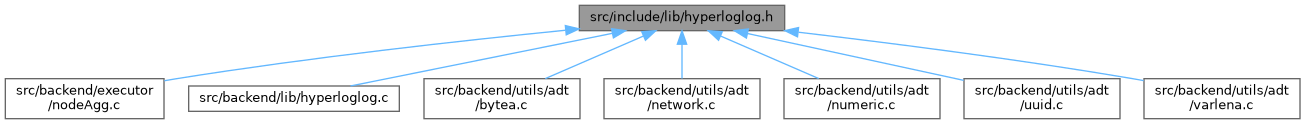PostgreSQL Source Code  git master
hyperloglog.h File Reference
This graph shows which files directly or indirectly include this file:Go to the source code of this file.

## Data Structures

struct  hyperLogLogState

## Typedefs

typedef struct hyperLogLogState hyperLogLogState

## Functions

void initHyperLogLog (hyperLogLogState *cState, uint8 bwidth)

void initHyperLogLogError (hyperLogLogState *cState, double error)

void addHyperLogLog (hyperLogLogState *cState, uint32 hash)

double estimateHyperLogLog (hyperLogLogState *cState)

void freeHyperLogLog (hyperLogLogState *cState)

## ◆ hyperLogLogState

 typedef struct hyperLogLogState hyperLogLogState

## Function Documentation

 void addHyperLogLog ( hyperLogLogState * cState, uint32 hash )

Definition at line 167 of file hyperloglog.c.

168 {
169  uint8 count;
170  uint32 index;
171
172  /* Use the first "k" (registerWidth) bits as a zero based index */
173  index = hash >> (BITS_PER_BYTE * sizeof(uint32) - cState->registerWidth);
174
175  /* Compute the rank of the remaining 32 - "k" (registerWidth) bits */
176  count = rho(hash << cState->registerWidth,
177  BITS_PER_BYTE * sizeof(uint32) - cState->registerWidth);
178
179  cState->hashesArr[index] = Max(count, cState->hashesArr[index]);
180 }
unsigned int uint32
Definition: c.h:495
#define Max(x, y)
Definition: c.h:987
unsigned char uint8
Definition: c.h:493
static uint8 rho(uint32 x, uint8 b)
Definition: hyperloglog.c:242
#define BITS_PER_BYTE
static unsigned hash(unsigned *uv, int n)
Definition: rege_dfa.c:715
uint8 * hashesArr
Definition: hyperloglog.h:58
Definition: type.h:95

## ◆ estimateHyperLogLog()

 double estimateHyperLogLog ( hyperLogLogState * cState )

Definition at line 186 of file hyperloglog.c.

187 {
188  double result;
189  double sum = 0.0;
190  int i;
191
192  for (i = 0; i < cState->nRegisters; i++)
193  {
194  sum += 1.0 / pow(2.0, cState->hashesArr[i]);
195  }
196
197  /* result set to "raw" HyperLogLog estimate (E in the HyperLogLog paper) */
198  result = cState->alphaMM / sum;
199
200  if (result <= (5.0 / 2.0) * cState->nRegisters)
201  {
202  /* Small range correction */
203  int zero_count = 0;
204
205  for (i = 0; i < cState->nRegisters; i++)
206  {
207  if (cState->hashesArr[i] == 0)
208  zero_count++;
209  }
210
211  if (zero_count != 0)
212  result = cState->nRegisters * log((double) cState->nRegisters /
213  zero_count);
214  }
215  else if (result > (1.0 / 30.0) * POW_2_32)
216  {
217  /* Large range correction */
218  result = NEG_POW_2_32 * log(1.0 - (result / POW_2_32));
219  }
220
221  return result;
222 }
#define POW_2_32
Definition: hyperloglog.c:54
#define NEG_POW_2_32
Definition: hyperloglog.c:55
int i
Definition: isn.c:73

## ◆ freeHyperLogLog()

 void freeHyperLogLog ( hyperLogLogState * cState )

Definition at line 151 of file hyperloglog.c.

152 {
153  Assert(cState->hashesArr != NULL);
154  pfree(cState->hashesArr);
155 }
Assert(fmt[strlen(fmt) - 1] !='\n')
void pfree(void *pointer)
Definition: mcxt.c:1456

References Assert(), hyperLogLogState::hashesArr, and pfree().

Referenced by hashagg_spill_finish().

## ◆ initHyperLogLog()

 void initHyperLogLog ( hyperLogLogState * cState, uint8 bwidth )

Definition at line 66 of file hyperloglog.c.

67 {
68  double alpha;
69
70  if (bwidth < 4 || bwidth > 16)
71  elog(ERROR, "bit width must be between 4 and 16 inclusive");
72
73  cState->registerWidth = bwidth;
74  cState->nRegisters = (Size) 1 << bwidth;
75  cState->arrSize = sizeof(uint8) * cState->nRegisters + 1;
76
77  /*
78  * Initialize hashes array to zero, not negative infinity, per discussion
79  * of the coupon collector problem in the HyperLogLog paper
80  */
81  cState->hashesArr = palloc0(cState->arrSize);
82
83  /*
84  * "alpha" is a value that for each possible number of registers (m) is
85  * used to correct a systematic multiplicative bias present in m ^ 2 Z (Z
86  * is "the indicator function" through which we finally compute E,
87  * estimated cardinality).
88  */
89  switch (cState->nRegisters)
90  {
91  case 16:
92  alpha = 0.673;
93  break;
94  case 32:
95  alpha = 0.697;
96  break;
97  case 64:
98  alpha = 0.709;
99  break;
100  default:
101  alpha = 0.7213 / (1.0 + 1.079 / cState->nRegisters);
102  }
103
104  /*
105  * Precalculate alpha m ^ 2, later used to generate "raw" HyperLogLog
106  * estimate E
107  */
108  cState->alphaMM = alpha * cState->nRegisters * cState->nRegisters;
109 }
size_t Size
Definition: c.h:594
#define ERROR
Definition: elog.h:39
void * palloc0(Size size)
Definition: mcxt.c:1257

## ◆ initHyperLogLogError()

 void initHyperLogLogError ( hyperLogLogState * cState, double error )

Definition at line 128 of file hyperloglog.c.

129 {
130  uint8 bwidth = 4;
131
132  while (bwidth < 16)
133  {
134  double m = (Size) 1 << bwidth;
135
136  if (1.04 / sqrt(m) < error)
137  break;
138  bwidth++;
139  }
140
141  initHyperLogLog(cState, bwidth);
142 }
void initHyperLogLog(hyperLogLogState *cState, uint8 bwidth)
Definition: hyperloglog.c:66
static void error(void)
Definition: sql-dyntest.c:147

References error(), and initHyperLogLog().